# Understanding zero divisors & ##\mathbb{Z_m}## in Ring Theory

• I
Gold Member
Homework Statement:: See attached
Relevant Equations:: Ring Theory

Trying to go through my undergraduate notes on Ring Theory ( in appreciation to my Professor who opened me up to the beautiful World of Math)...anyways see attached...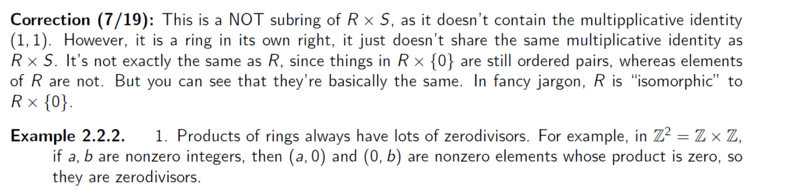I need some clarity on the zero divisor. I am aware that we may have a left or right zero divisor where ##a## is an element in a ring ##R## and there exists a non zero ##x## in ##R## such that,
##ax=0##, now looking at the above example, 2.2.2 we have ##(a,0)⋅(0,b)=(a⋅0, 0⋅b)=(0,0)##, since product is equal to ##(0,0)## then we conclude that the elements are zero divisors. Now my question is, using the definition, ##ax=0##, and given that ##a,b## are elements in ##R##, then what would be our ##x?##
or are we supposed to say, consider ##(a,0)## as our ##a## and ##(0,b)## as our ##x##...cheers

I think i get it, ##(a,0)## is an element in ##R## and ##(0,b)## is the non zero element in ##R##. Right, let me continue with looking at the literature, i will ask questions in areas of doubt.

Last edited:

Gold Member
Hello kindly i need insight here, on the highlighted part...and how they arrived at the conclusion whereas we have many values that satisfy the integer modulo ##m=4##.
I think i understand the concept applied i.e
Let ##m>0##, we define the integer ##b##,

##b=\left\{x:x≡b \mod m\right\}##

I need clarity or rather reasons as to why they picked the indicated values...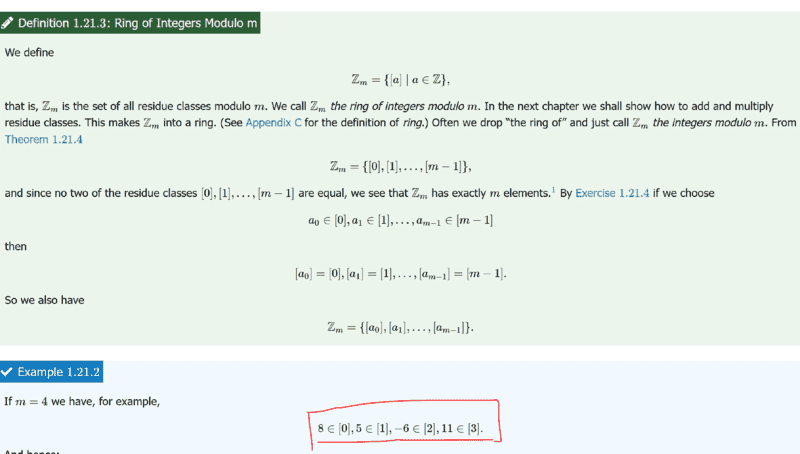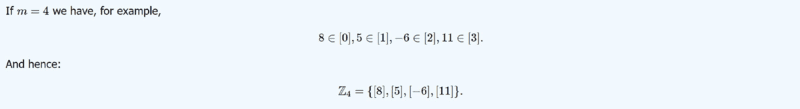From my own analysis, for ##\mathbb{Z_4}## we shall have
##[a_0]= ...-4, 0, 4, 8, 12, ...##
##[a_1]= ...-3, 1, 5, 9, 13, ...##
##[a_2]= ...-6,-2, 2, 6, 10, 14, ...##
##[a_3]= ...-5,-1, 3, 7, 11, 15, ...##

Is there any particular reason as to why they picked ##8,5, -6## and ##11## or we could pick any values from the sets?

Last edited by a moderator:
1. Difficult to follow. Please try to express yourself more concisely. If necessary, highlight the part in your posts that contain the questions.
2. This is not a homework question.

Gold Member
OK before i proceed i would like to know if it is allowed to explore 'difficult'concepts here or it is only homework questions which are allowed. My opening remarks was to try and familiarise with some areas on Ring theory. If this is not allowed do let me know...having said that i was looking at;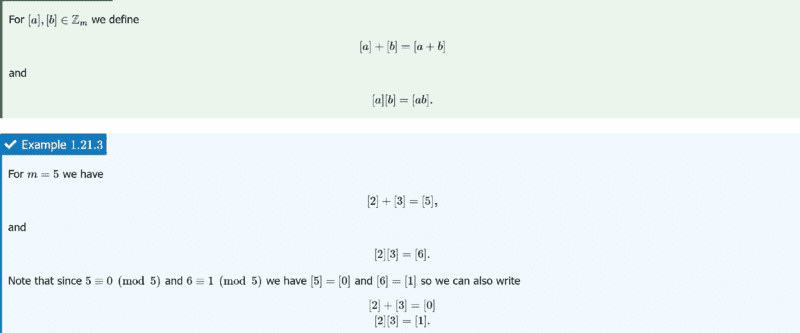Do note that we are looking at Ring of Integers Modulo m...for the posts 2 to 4.

From the attachment (in post 4), i noted that they used, ##[a] =[a_2]## and
b=##[a_3]## ...now are my steps correct? if i would say use,

##[a_0]=15## and ##[a_4] = 24## to realize,
####+#### = ####
##⋅ ##=##360## to therefore have,
####+#### = ####
##⋅ ##=##0##. Thanks

Last edited:
Gold Member
1. Difficult to follow. Please try to express yourself more concisely. If necessary, highlight the part in your posts that contain the questions.
2. This is not a homework question.
Noted ...the part circled by myself in red is the area of interest in post 2. Note that,
##8∈[a_0]##my question is could we as well have picked any other values in ##[a_0]## to satisfy the conclusion made or is there a specific reason as to why ##8## was picked? ##[a_0]## has other elements like indicated i.e
##[a_0]= ...-4, 0, 4, 8, 12, ...##

The computations are carried out in ##\mathbb Z_m##, not ##\mathbb Z##. So, the same conclusion follows if you use arbitrary representatives from the respective congruence classes.

For example in ##\mathbb Z_{7}##, ## +  =  ## is equivalent to ##  + [-5] =  ##.

It is not true that ##[a_0] = \ldots -4, 0,4\ldots##. It is true that ##[a_0] = \{\ldots, -4,0,4\ldots\} = \{a\in\mathbb Z \mid a\equiv a_0 \pmod{m}\} ##.

•chwala
Gold Member
The computations are carried out in ##\mathbb Z_m##, not ##\mathbb Z##. So, the same conclusion follows if you use arbitrary representatives from the respective congruence classes.

For example in ##\mathbb Z_{7}##, ## +  =  ## is equivalent to ##  + [-5] =  ##.

It is not true that ##[a_0] = \ldots -4, 0,4\ldots##. It is true that ##[a_0] = \{\ldots, -4,0,4\ldots\} = \{a\in\mathbb Z \mid a\equiv a_0 \pmod{m}\} ##.
Thanks I just missed out on the bracket...but my intention is of course what you have indicated... I will endeavour to be careful with the notations though.. thanks @nuuskur.

Gold Member
Imagine you're dealing with a money system based on cents, so that 100 cents equals one unit. Then 100 becomes your new 0. So does every multiple of 100. Then:
25(4)=50(2)=100=0.

•chwala
Gold Member
The computations are carried out in ##\mathbb Z_m##, not ##\mathbb Z##. So, the same conclusion follows if you use arbitrary representatives from the respective congruence classes.

For example in ##\mathbb Z_{7}##, ## +  =  ## is equivalent to ##  + [-5] =  ##.

It is not true that ##[a_0] = \ldots -4, 0,4\ldots##. It is true that ##[a_0] = \{\ldots, -4,0,4\ldots\} = \{a\in\mathbb Z \mid a\equiv a_0 \pmod{m}\} ##.
##\mathbb Z_{7}##, ## +  =  ## is equivalent to (in terms of ##[a_m]## notation);
##[a_3]+[a_2]=  +  =  ##
##[a_{17}]+[a_2]=  + [-5] =  ##

Last edited:
Staff Emeritus
Homework Helper
Gold Member
OK before i proceed i would like to know if it is allowed to explore 'difficult' concepts here or it is only homework questions which are allowed. My opening remarks was to try and familiarise with some areas on Ring theory. If this is not allowed do let me know...having said that i was looking at;

View attachment 299690
A moderator moved this thread from "Calculus & Beyond" Homework to this Forum, so this thread is allowed here.

Try to be more focused with your questions. That's not always easy when you are somewhat confused about the topics you're unclear about.

•chwala
Gold Member
A moderator moved this thread from "Calculus & Beyond" Homework to this Forum, so this thread is allowed here.

Try to be more focused with your questions. That's not always easy when you are somewhat confused about the topics you're unclear about.
Noted Sammy. Cheers.

Gold Member
Still progressing with study, i want to to be certain am getting this right. Consider the example below;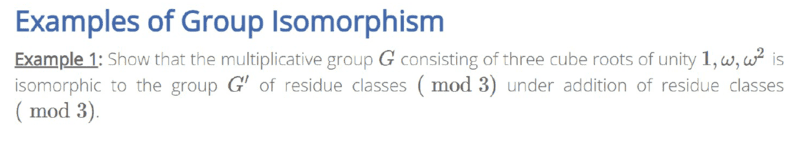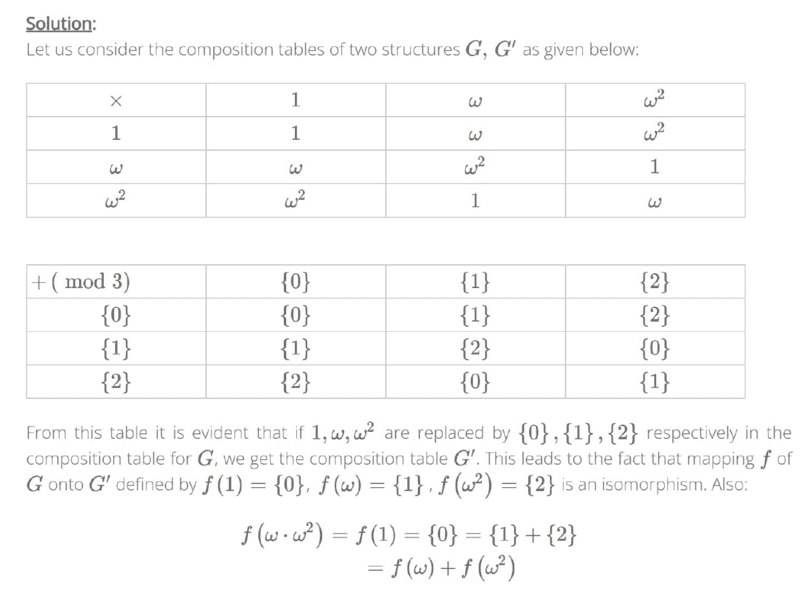The steps are pretty straightforward to me. The thing to note is that for isomorphism ( The ring homomorphism has to be a 1-1 and onto). with the condition,
##f(x+y) = f(x)+f(y)##
##f(xy)=f(x)f(y)##, ∀x,y∈##\mathbb{R}##

Now allow me create my own question here following the above example but for (mod 4).

 × ##1## ##w## ##w^2## ##w^3## ##1## ##1## ##w## ##w^2## ##w^3## ##w## ##w## ##w^2## ##w^3## ##1## ##w^2## ##w^2## ##w^3## ##1## ##w## ##w^3## ##w^3## ##1## ##w## ##w^2##​

 + (mod 4) ##0## ##1## ##2## ##3## ##0## ##0## ##1## ##2## ##3## ##1## ##1## ##2## ##3## ##0## ##2## ##2## ##3## ##0## ##1## ##3## ##3## ##0## ##1## ##2##

##f(1)=0## , ##f(w)=1##, ##f(w^2)=2##, ##f(w^3)=3## thus is an isomorphism.
Also,
##f(w^3⋅w^2)=f(w)=1=+=f(w^3)+f(w^2)##... just sharing in case of any feedback...meanwhile i will continue studying on this...really interesting area...

Last edited:
•nuuskur
Gold Member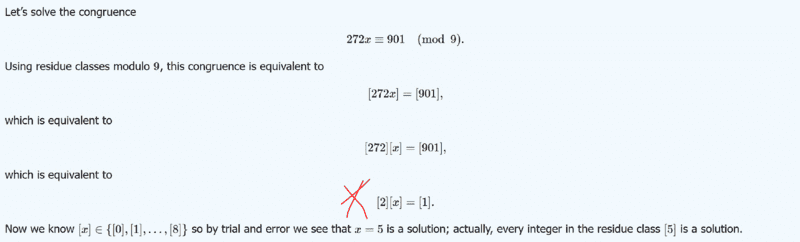I think text has mistake on highlighted part, it ought to be,
##[x]=## then as they say using trial and error method .There could be a better way of solving such equations ...i would need to check...the residue class #### has ##\{\ldots, -4,5,14,23, \ldots\}## any of the values would satisfy the congruence equation.

...unless they applied mod 9 on both sides of equation to then end up with the equation##[x]=## ...if that is the case then the equation would hold...

Last edited:
Staff Emeritus
Homework Helper
Gold Member
View attachment 299736

I think text has mistake on highlighted part, it ought to be,
##[x]=## then as they say using trial and error method .There could be a better way of solving such equations ...i would need to check...the residue class #### has ##\{\ldots, -4,5,14,23, \ldots\}## any of the values would satisfy the congruence equation.

...unless they applied mod 9 on both sides of equation to then end up with the equation##[x]=## ...if that is the case then the equation would hold...
No mistake in the text. (However, your graphic image is barely readable .)

Mathematically the term (as in terminology) "mod" has more than one meaning, The meanings are related and depend on context. The concept is also related to the term "modulo".

As a mathematical operator/function you might say: 272 mod 9 = 1 .
Basically this gives the remainder of integer division for 272 divided by 9 as being 1 .

What you're referenced text refers to is somewhat different.

(Added in Edit: After zooming in on the graphic I see that indeed an equivalence sign is used there, so I am changing this post consistent with this. )
For the statement:
##272x\equiv 901 \text{ mod } 9## , ( Edited )
you might say ##272## times ##x## is congruent to ##901## mod ##9## . Often you will see the "equivalent sign", ##\equiv##, used rather than an "equal sign".

Of course, with residue classes this is written as: ##[272x]=##

I must make the observation that you have this thread jumping all over the place - topic-wise.

You have noted somewhere above that ##[a+b]=[a]+[ b]## .
In a ring, a similar result holds for the multiplication operation: ##[a\cdot b]=[a]\cdot [ b]##.

Therefore, it is correct to say that it follows that:

##[x]=##

So of course for residue classes mod 9 we have, ##[x]=##

Last edited:
•chwala
Gold Member
Thanks Sammy...I think way forward is for me to have new posts (open new threads) to avoid ' mixing up' the posts in question...well noted.

•SammyS
Staff Emeritus
Homework Helper
Gold Member
Speaking of residue classes modulo 9:

What are the zero divisors in the ring ##\mathbb{Z}_{~9} ~ ?##

Gold Member
Speaking of residue classes modulo 9:

What are the zero divisors in the ring ##\mathbb{Z}_{~9} ~ ?##
If i understand your question we are looking for ##xy≡0 mod 9## with the condition that ##x≠0 mod 9##and ##y≠0 mod 9##,
Now we have,
##[a_0]##=##\{\ldots, -9,0,9,18,27 \ldots\}##
##[a_1]##=##\{\ldots, -8,1,10,19,28 \ldots\}##
if we pick ##[a_0]##=##9## and ##[a_1]##=##1##, then ##[a_0]⋅[a_1]=9⋅1=0 mod 9##

just a minute i check ##1## cannot be a zero divisor in any ring!

Second attempt.

##[a_0]##=##\{\ldots, -9,0,9,18,27 \ldots\}##
##[a_2]##=##\{\ldots, -7,2,11,20,29 \ldots\}##
if we pick ##[a_0]##=##9## and ##[a_2]##=##2##, then ##[a_0]⋅[a_2]=9⋅2=0 mod 9## therefore ##9## and ##2## are zero divisors.

@chwala Few golden rules to remember in modular arithmetic.
1. Any non-zero element in a finite ring is either a zero divisor or invertible.
2. An element ##[a]\in\mathbb Z_m## is invertible if and only if ##\mathrm{gcd}(a,m)=1##.

•chwala and WWGD
Gold Member
@chwala Few golden rules to remember in modular arithmetic.
1. Any non-zero element in a finite ring is either a zero divisor or invertible.
2. An element ##[a]\in\mathbb Z_m## is invertible if and only if ##\mathrm{gcd}(a,m)=1##.
Maybe add that for ##p## prime, every element in ##\mathbb Z _p## is invertible and the ring is a Field.

•chwala
Gold Member
@chwala Few golden rules to remember in modular arithmetic.
1. Any non-zero element in a finite ring is either a zero divisor or invertible.
2. An element ##[a]\in\mathbb Z_m## is invertible if and only if ##\mathrm{gcd}(a,m)=1##.
Thanks @nuuskur...with no zero divisors we can look at this in terms of integral domain. Right?

Thanks @nuuskur...with no zero divisors we can look at this in terms of integral domain. Right?
I am not entirely sure, what you mean. Integral domains are commutative rings with identity that contain no zero divisors, i.e, ##xy=0## implies either ##x=0## or ##y=0##. For example, any field is an integral domain.

Gold Member
I am not entirely sure, what you mean. Integral domains are rings with identity that contain no zero divisors, i.e, ##xy=0## implies either ##x=0## or ##y=0##. For example, any field is an integral domain.
I meant that if a Ring has no zero divisors then the Ring is an Integral domain...unless i am getting it wrong ...still familiarising on the literature.

Gold Member
unless i got Sammy wrong, i think what he meant was,
##[272 mod 9] [x]≡ 901 mod 9##
##[272 mod 9] [x]≡ 1##
We know that ##272 mod 9 =2##
then we shall therefore have ##[x]=##

I meant that if a Ring has no zero divisors then the Ring is an Integral domain...
I slipped up. Has to be a commutative ring. And no, a ring need not have a multiplicative identity. It has to be a commutative ring with identity.

Gold Member
I slipped up. Has to be a commutative ring. And no, a ring need not have a multiplicative identity. It has to be a commutative ring with identity.
Thanks let me read more on this...i am more inclined towards applied Maths...but i find pure Math quite interesting...let me read more on this..a different world altogether.

Gold Member
Thanks let me read more on this...i am more inclined towards applied Maths...but i find pure Math quite interesting...let me read more on this..a different world altogether.
An example of a non-commutative ring with identity is that of the nxn matrices over the Reals ( using entries on a field to simplify). They're not an integral domain.

•chwala
Gold Member
Imagine the group of rotations. Then the trivial rotation is by 0 degrees. What happens if you do four rotations by $$\pi/2=90^0$$ degrees? Or 6 rotations , each by $$2pi/6=60^0$$ degrees?

Gold Member
Imagine the group of rotations. Then the trivial rotation is by 0 degrees. What happens if you do four rotations by $$\pi/2=90^0$$ degrees? Or 6 rotations , each by $$2pi/6=60^0$$ degrees?
Hey @WWGD are we looking at cyclic groups or what? Just mention the area then i will check this up...

Maarten Havinga
##f(1)=0## , ##f(w)=1##, ##f(w^2)=2##, ##f(w^3)=3## thus is an isomorphism.
Also,
##f(w^3⋅w^2)=f(w)=1=+=f(w^3)+f(w^2)##... just sharing in case of any feedback...meanwhile i will continue studying on this...really interesting area...
You're right!
if we pick ##[a_0]##=##9## and ##[a_2]##=##2##, then ##[a_0]⋅[a_2]=9⋅2=0 mod 9## therefore ##9## and ##2## are zero divisors.
Good try! But modulo 9, ##=## is the zero element so these are not examples of zero divisors. Try again!

•chwala
Maarten Havinga
Hey @WWGD are we looking at cyclic groups or what? Just mention the area then i will check this up...
Although I understand why you react this way, rotations in 2D do happen to be cyclic groups.

Maarten Havinga
I think text has mistake on highlighted part, it ought to be,
##[x]=## then as they say using trial and error method .

...unless they applied mod 9 on both sides of equation to then end up with the equation##[x]=## ...if that is the case then the equation would hold...
Your unless statement is right on the mark. The idea is that modulo 9 any representative of one of the equivalence classes #### up to #### is the same element. So ##===[-7]=...##. Writing ##x=## is therefore equivalent to ##x=## since ##272=2## and ##901=1## modulo 9.

•chwala
Gold Member
Hey @WWGD are we looking at cyclic groups or what? Just mention the area then i will check this up...
Actually, no, these are cyclic subgroups of the group of all rotations. This group of all rotations is uncountable and uncountable groups cannot be cyclic.

Gold Member
Although I understand why you react this way, rotations in 2D do happen to be cyclic groups.
Not sure what you mean. Say you have a circle in the plane. Then you can rotate it by any of the uncountable many amounts in $$[0, 2\pi]$$. This is an uncountable set and thus the group cannot be cyclic.

Gold Member
You're right!

Good try! But modulo 9, ##=## is the zero element so these are not examples of zero divisors. Try again!

You're right!

Good try! But modulo 9, ##=## is the zero element so these are not examples of zero divisors. Try again!

Ok, i see your point we cannot pick any item in ##\left [a_0\right]##, in that case we ought to have;

##⋅= 0 mod 9##
##⋅= 0 mod 9##
##⋅= 0 mod 9##

I can also see that,
##⋅= 1 mod 9## implying that #### is an inverse of ####, same to ##7## and ##4##...

•Maarten Havinga
Maarten Havinga
Not sure what you mean. Say you have a circle in the plane. Then you can rotate it by any of the uncountable many amounts in $$[0, 2\pi]$$. This is an uncountable set and thus the group cannot be cyclic.
That's a matter of definition IMO. But you're technically right.
If we include exponentials in generated groups ofgenerator sets and allow limits of fractional exponentials if they're in the group, your circle is generated by a single rotation.